1. /
2. CBSE
3. /
4. Class 12
5. /
6. Physics
7. /
8. NCERT Solutions class 12...

# NCERT Solutions class 12 physics Electrostatic Potential and Capacitance### myCBSEguide App

Download the app to get CBSE Sample Papers 2023-24, NCERT Solutions (Revised), Most Important Questions, Previous Year Question Bank, Mock Tests, and Detailed Notes.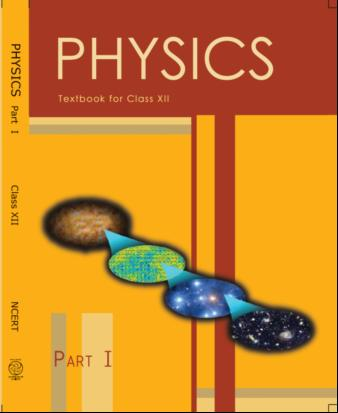## NCERT Class 12 Physics Chapter-wise Solutions

• 1 – Electric Charges and Fields
• 2 – Electrostatic Potential and Capacitance
• 3 – Current Electricity
• 4 – Moving Charges and Magnetism
• 5 – Magnetism and Matter
• 6 – Electromagnetic Induction
• 7 – Alternating Current
• 8 – Electromagnetic Waves
• 9 – Ray Optics and Optical Instruments
• 10 – Wave Optics
• 11 – Dual Nature of Radiation and Matter
• 12 – Atoms
• 13 – Nuclei
• 14 – Semiconductor Electronic: Material, Devices and Simple Circuits
• 15 – Communication Systems

## CHAPTER 2 ELECTROSTATIC POTENTIAL AND CAPACITANCE

• 2.1 Introduction
• 2.2 Electrostatic Potential
• 2.3 Potential due to a Point Charge
• 2.4 Potential due to an Electric Dipole
• 2.5 Potential due to a System of Charges
• 2.6 Equipotential Surfaces
• 2.7 Potential Energy of a System of Charges
• 2.8 Potential Energy in an External Field
• 2.9 Electrostatics of Conductors
• 2.10 Dielectrics and Polarisation
• 2.11 Capacitors and Capacitance
• 2.12 The Parallel Plate Capacitor
• 2.13 Effect of Dielectric on Capacitance
• 2.14 Combination of Capacitors
• 2.15 Energy Stored in a Capacitor
• 2.16 Van de Graaff Generator

## NCERT Solutions class 12 physics Electrostatic Potential and Capacitance Part 1

1:   Two charges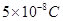and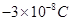are located 16 cm apart. At what point(s) on the line joining the two charges is the electric potential zero? Take the potential at infinity to be zero.

2.  A regular hexagon of side 10 cm has a charge 5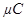at each of its vertices. Calculate the potential at the centre of the hexagon.

3:  Two charges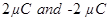are placed at points A and B 6 cm apart.

i. Identify an equipotential surface of the system.

ii. What is the direction of the electric field at every point on this surface?

4:  A spherical conductor of radius 12 cm has a charge of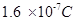distributed uniformly on its surface. What is the electric field

i. Inside the sphere

ii. Just outside the sphere

iii. At a point 18 cm from the centre of the sphere?

5:  A parallel plate capacitor with air between the plates has a capacitance of 8 pF (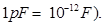What will be the capacitance if the distance between the plates is reduced by half, and the space between them is filled with a substance of dielectric constant 6?

6:  Three capacitors each of capacitance 9 pF are connected in series.

i. What is the total capacitance of the combination?

ii. What is the potential difference across each capacitor if the combination is connected to a 120 V supply?

7:  Three capacitors of capacitances 2 pF, 3 pF and 4 pF are connected in parallel.

i. What is the total capacitance of the combination?

ii. Determine the charge on each capacitor if the combination is connected to a 100 V supply.

8:  In a parallel plate capacitor with air between the plates, each plate has an area of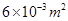and the distance between the plates is 3 mm. Calculate the capacitance of the capacitor. If this capacitor is connected to a 100 V supply, what is the charge on each plate of the capacitor?

9:  Explain what would happen if in the capacitor given in Exercise 2.8, a 3 mm thick mica sheet (of dielectric constant = 6) were inserted between the plates,

i. While the voltage supply remained connected.

2. After the supply was disconnected.

10:  A 12 pF capacitor is connected to a 50V battery. How much electrostatic energy is stored in the capacitor?

11. A 600 pF capacitor is charged by a 200 V supply. It is then disconnected from the supply and is connected to another uncharged 600 pF capacitor. How much electrostatic energy is lost in the process?

12: A charge of 8 mC is located at the origin. Calculate the work done in taking a small charge of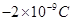from a point P (0, 0, 3 cm) to a point Q (0, 4 cm, 0), via a point R (0, 6 cm, 9 cm).

13: A cube of side b has a charge q at each of its vertices. Determine the potential and electric field due to this charge array at the centre of the cube.

14: Two tiny spheres carrying charges 1.5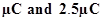are located 30 cm apart. Find the potential and electric field:

i. at the mid-point of the line joining the two charges, and

ii. at a point 10 cm from this midpoint in a plane normal to the line and passing through the mid-point.

15: A spherical conducting shell of inner radius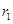and outer radius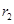has a charge

1. A charge q is placed at the centre of the shell. What is the surface charge density on the inner and outer surfaces of the shell?

2. Is the electric field inside a cavity (with no charge) zero, even if the shell is not spherical, but has any irregular shape? Explain.

16:  Show that the normal component of electrostatic field has a discontinuity from one side of a charged surface to another given by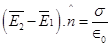Where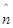is a unit vector normal to the surface at a point and Ïƒ is the surface charge density at that point. (The direction ofis from side 1 to side 2.) Hence show that just outside a conductor, the electric field is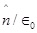1. Show that the tangential component of electrostatic field is continuous from one side of a charged surface to another. [Hint: For (a), use Gauss’s law. For, (b) use the fact that work done by electrostatic field on a closed loop is zero.]

17: A long charged cylinder of linear charged density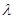is surrounded by a hollow co-axial conducting cylinder. What is the electric field in the space between the two cylinders?

18: In a hydrogen atom, the electron and proton are bound at a distance of about 0.53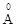.

1. Estimate the potential energy of the system in eV, taking the zero of the potential energy at infinite separation of the electron from proton.

2. What is the minimum work required to free the electron, given that its kinetic energy in the orbit is half the magnitude of potential energy obtained in (a)?

3. What are the answers to (a) and (b) above if the zero of potential energy is taken at 1.06separation?

19: If one of the two electrons of a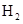molecule is removed, we get a hydrogen molecular ion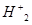. In the ground state of an, the two protons are separated by roughly 1.5, and the electron is roughly 1from each proton.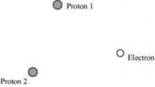## NCERT Solutions for Class 12 Physics

NCERT Solutions Class 12 Physics PDF (Download) Free from myCBSEguide app and myCBSEguide website. Ncert solution class 12 physics includes text book solutions from both part 1 and part 2. NCERT Solutions for CBSE Class 12 Physics have total 20 chapters. 12 Physics NCERT Solutions in PDF for free Download on our website. Ncert physics class 12 solutions PDF and physics ncert class 12 PDF solutions with latest modifications and as per the latest CBSE syllabus are only available in myCBSEguide

To download NCERT Solutions for class 12 Physics, Chemistry, Biology, History, Political Science, Economics, Geography, Computer Science, Home Science, Accountancy, Business Studies and Home Science; do check myCBSEguide app or website. myCBSEguide provides sample papers with solution, test papers for chapter-wise practice, NCERT solutions, NCERT Exemplar solutions, quick revision notes for ready reference, CBSE guess papers and CBSE important question papers. Sample Paper all are made available through the best app for CBSE students and myCBSEguide website.### Test Generator

Create question paper PDF and online tests with your own name & logo in minutes.### myCBSEguide

Question Bank, Mock Tests, Exam Papers, NCERT Solutions, Sample Papers, Notes